# Node.js web page controlled Robotic Car (Wireless)

A wireless ROVER which is controlled by a web-page served by Node.js server, with the help of Sockets.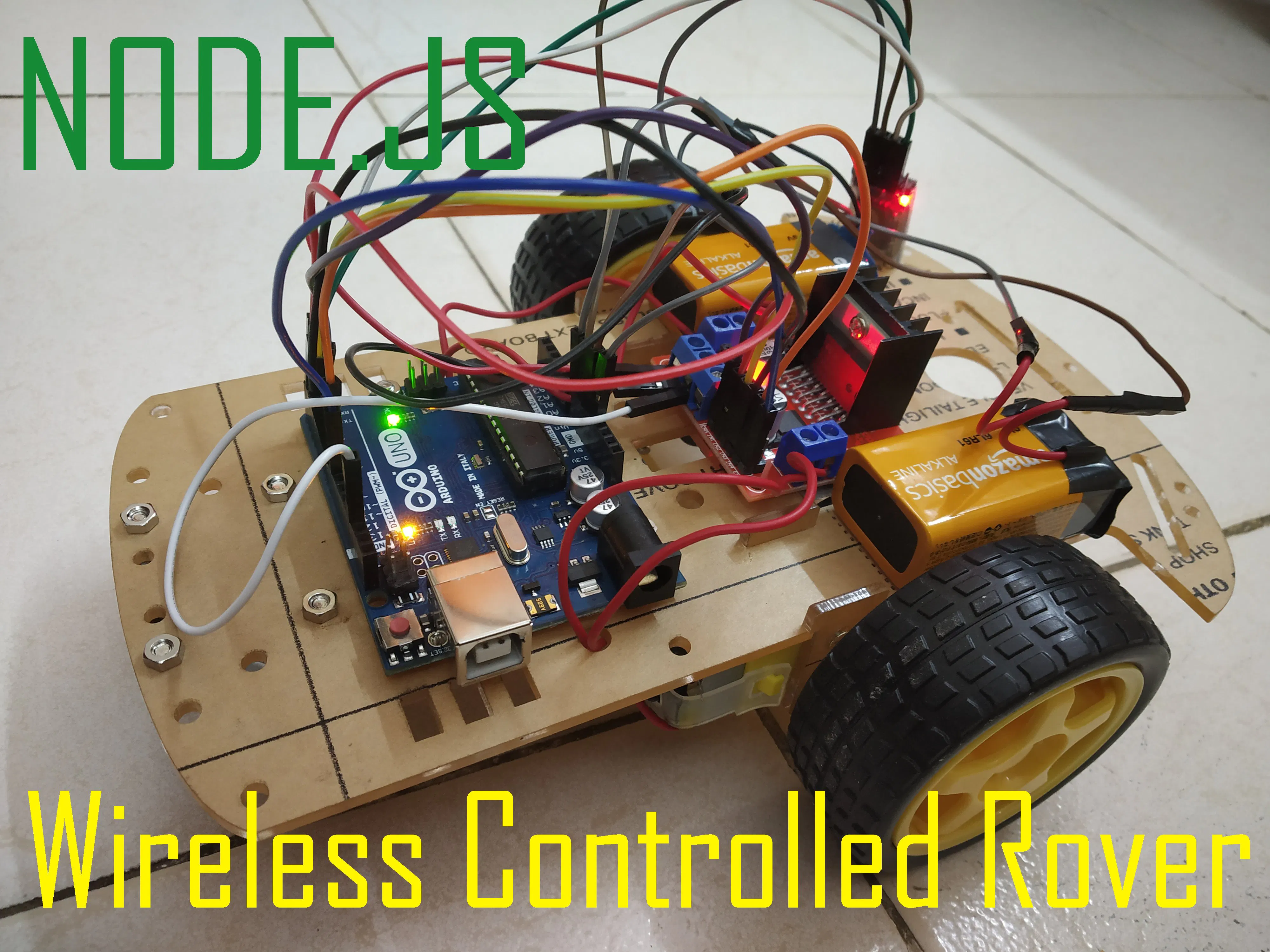## Things used in this project

### Hardware componentsArduino UNO
×1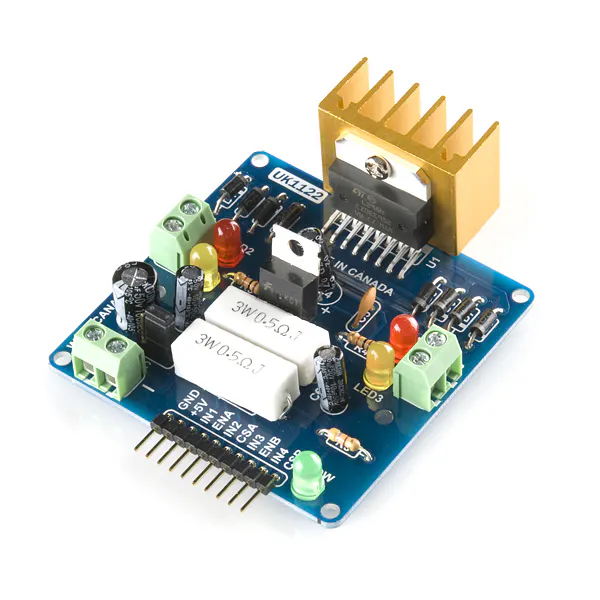SparkFun Dual H-Bridge motor drivers L298
×1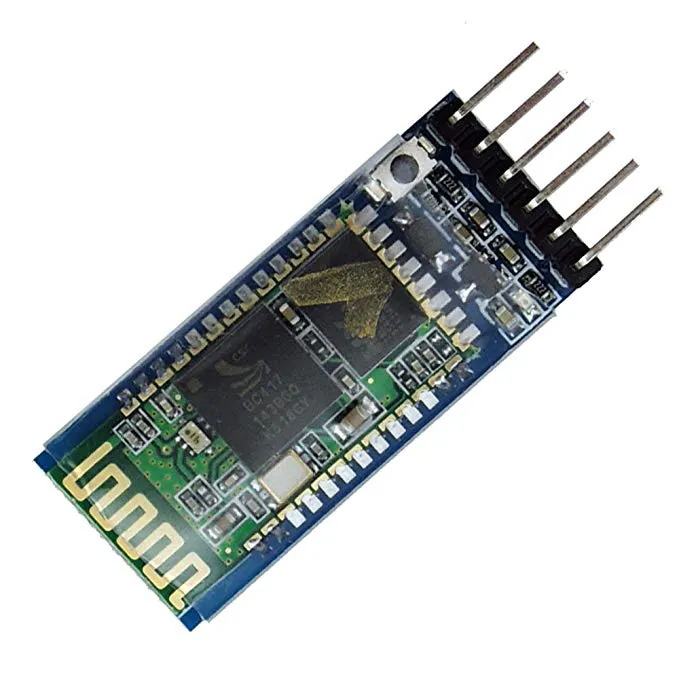HC-05 Bluetooth Module
×1Jumper wires (generic)
×1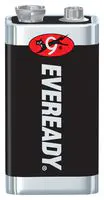Battery, 9 V
×2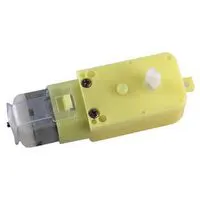DC Motor, 12 V
×2

## Schematics

### Circuit Diagram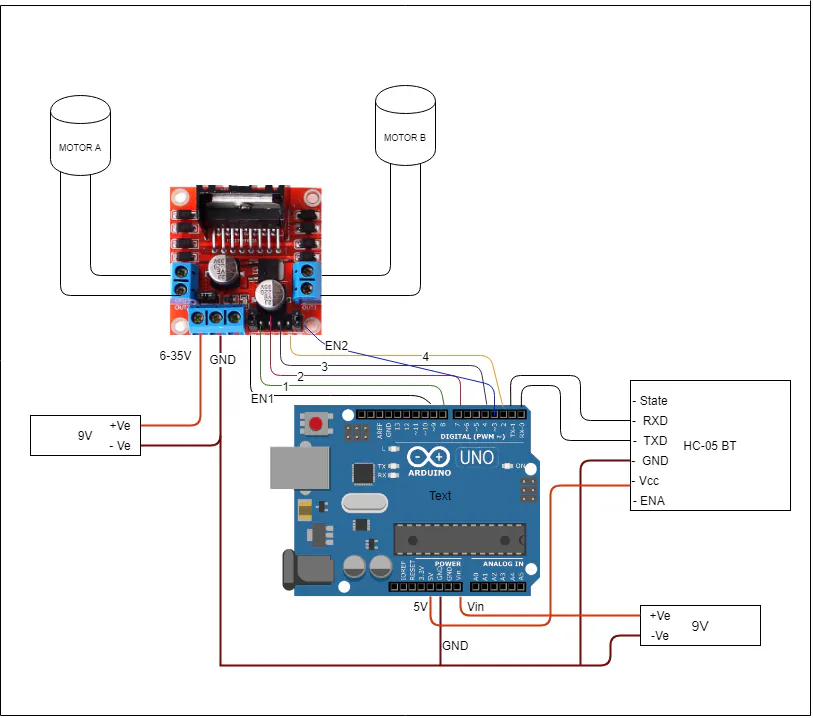### Data Transmission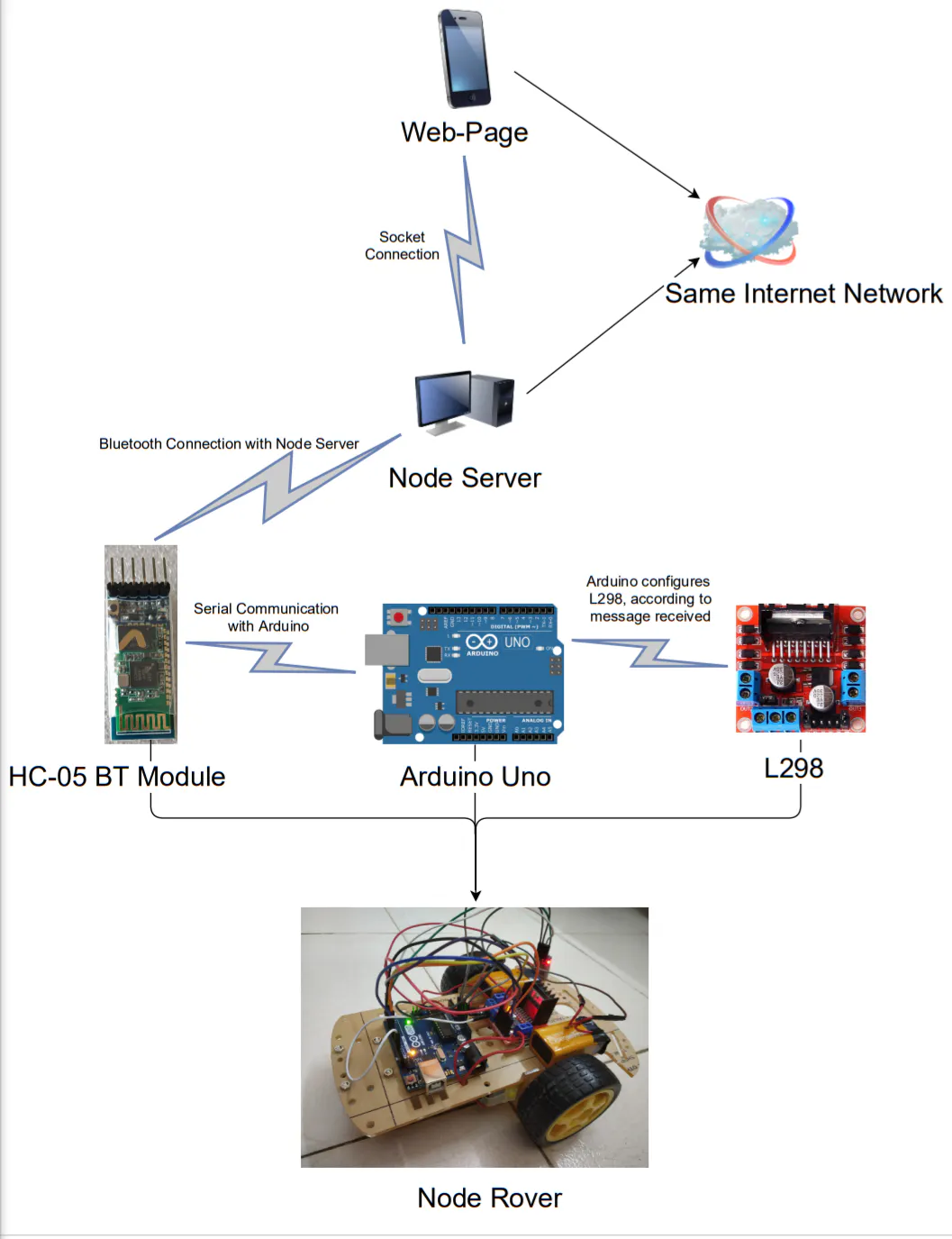## Code

### Node Rover Arduino Code

Arduino
```const int en1 = 9;
const int en2 = 3;
const int inp1 = 2;
const int inp2 = 4;
const int inp3 = 7;
const int inp4 = 8;
String inputString = "";

void setup() {
Serial.begin(9600);
pinMode(en1, OUTPUT);
pinMode(en2, OUTPUT);
pinMode(inp1, OUTPUT);
pinMode(inp2, OUTPUT);
pinMode(inp3, OUTPUT);
pinMode(inp4, OUTPUT);
}

void loop() {
// put your main code here, to run repeatedly:
if (Serial.available()) {
} else {
if (inputString == "forward") {
// To move Forward
digitalWrite(inp1, HIGH);
digitalWrite(inp2, LOW);
digitalWrite(inp3, HIGH);
digitalWrite(inp4, LOW);
} else if (inputString == "backward") {
// To move backward
digitalWrite(inp1, LOW);
digitalWrite(inp2, HIGH);
digitalWrite(inp3, LOW);
digitalWrite(inp4, HIGH);
} else if (inputString == "fleft") {
// To move left
digitalWrite(inp1, HIGH);
digitalWrite(inp2, LOW);
digitalWrite(inp3, LOW);
digitalWrite(inp4, LOW);
} else if (inputString == "fright") {
// To move right
digitalWrite(inp1, LOW);
digitalWrite(inp2, LOW);
digitalWrite(inp3, HIGH);
digitalWrite(inp4, LOW);
}
else if (inputString == "bleft") {
// To move back left
digitalWrite(inp1, LOW);
digitalWrite(inp2, LOW);
digitalWrite(inp3, LOW);
digitalWrite(inp4, HIGH);
}
else if (inputString == "bright") {
// To move back right
digitalWrite(inp1, LOW);
digitalWrite(inp2, HIGH);
digitalWrite(inp3, LOW);
digitalWrite(inp4, LOW);
}
else if (inputString == "break") {
// To Stop
analogWrite(en1, 50);
analogWrite(en2, 50);
delay(550);
digitalWrite(inp1, LOW);
digitalWrite(inp2, LOW);
digitalWrite(inp3, LOW);
digitalWrite(inp4, LOW);
analogWrite(en1, 150);
analogWrite(en2, 150);
}
else if (inputString == "maxspeed") {
// Max speed
analogWrite(en1, 250);
analogWrite(en2, 250);
}
else if (inputString == "medspeed") {
// Medium Speed
analogWrite(en1, 180);
analogWrite(en2, 180);
}
else if (inputString == "lowspeed") {
// Low speed
analogWrite(en1, 90);
analogWrite(en2, 90);
}
else {
// Stop
digitalWrite(inp1, LOW);
digitalWrite(inp2, LOW);
digitalWrite(inp3, LOW);
digitalWrite(inp4, LOW);
}
inputString = "";
}
}
}
```